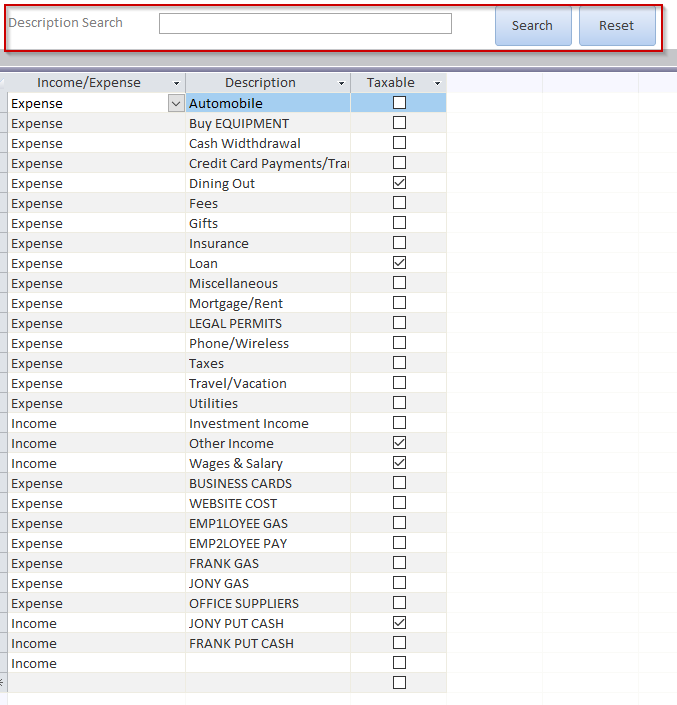# MS Access VBA Form Filter Example

In a previous post I gave an example of a MS Access form filter.

This revision is to demonstrate how to requery a form after the combobox changes, and not when a button is clicked.## Here is the code:

```Private Sub cboDescription_AfterUpdate()

Me.FilterOn = False
m_Where = m_Where & " AND Description = '" & Me.cboDescription & "'"
FilterThis

End Sub

Private Sub cboIncomeExpense_AfterUpdate()
Me.FilterOn = False

'clear the WHERE variable
m_Where = vbNullString

m_Where = m_Where & " AND [Income/Expense] = '" & Me.cboIncomeExpense & "'"
FilterThis
End Sub
Private Sub chkTax_AfterUpdate()
m_Where = m_Where & " AND [Taxable] = " & Me.chkTax
FilterThis
End Sub

Sub FilterThis()

'clean the string first
If Left(m_Where, 4) = " AND" Then
m_Where = Mid(m_Where, 5)
End If

Me.Filter = m_Where
Me.FilterOn = True

End Sub
```

## I also added a “search” box to query a table displayed in a lower form.## Here is the code:

```Private m_Where As String

Private Sub btnReset_Click()
Me.RecordSource = "Categories"

Me.FilterOn = False

'clear all the variables
m_Where = ""
Me.cboDescription = ""
Me.cboIncomeExpense = ""
Me.chkTax = 0

End Sub

Private Sub btnSearch_Click()
'Search as you type

Dim strSearch As String
Dim strSQL As String

strSQL = "SELECT * FROM Categories WHERE Description like """ & Me.txtSearch & "*" & """"

Me.RecordSource = strSQL

End Sub
```

Let me know if I need to elaborate.

#### “Go Fund Me” Page(\$5 suggested amount)## Free! Subscribe To Our YouTube Channel!